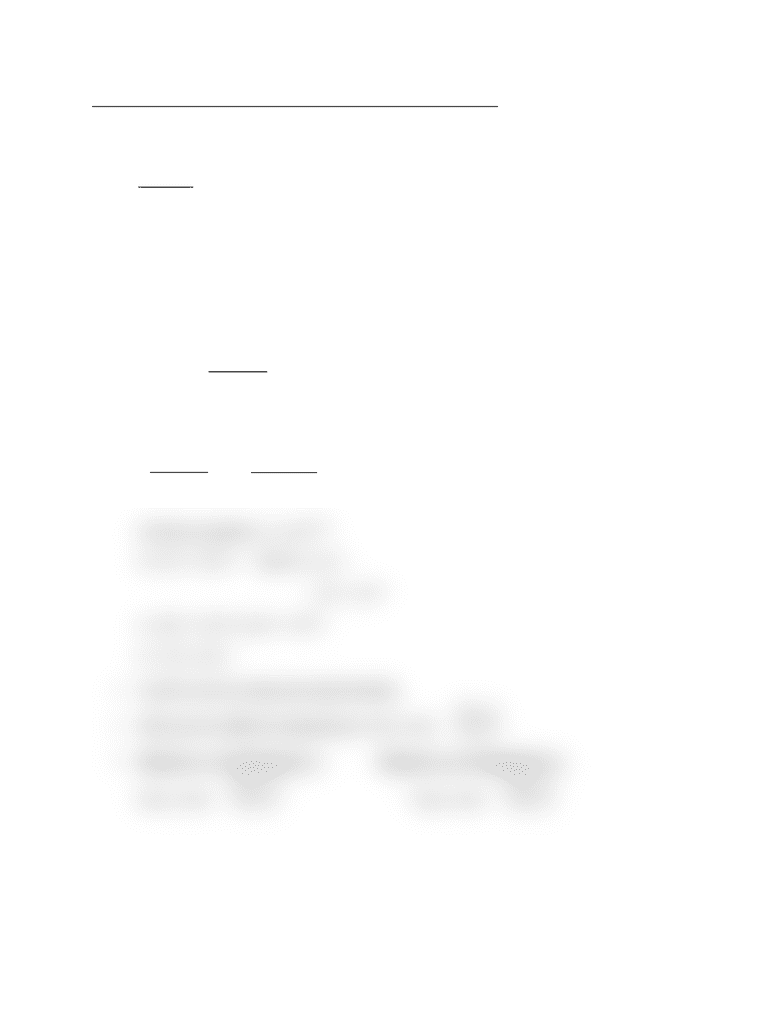Class Notes (1,100,000)
CA (620,000)
UTSG (50,000)
CHM (800)
CHM135H1 (400)
Lecture 17

# CHM135H1 Lecture Notes - Lecture 17: Reaction Rate Constant, Reaction Mechanism, ChlorofluorocarbonPremium

Department
Chemistry
Course Code
CHM135H1
Professor
Kris Quinlan
Lecture
17

This preview shows half of the first page. to view the full 2 pages of the document.23/02/16
CHM 139 – Lecture 17 – Effect of Temperature and Catalysts
Dependence of Rate upon Temperature:
Temperature dependence of rate constant, k, due (mostly) to f
Example
Recall: 25°C 35°C = 3 × rate
Net: NO(g) + O3(g) NO2(g) + O2(g) Ea = 63 kJ/mol
f (25°C) = e-Ea/RT f (35°C) = e-Ea/RT
= e-63000Jmol-1/R(298K) = e-63000Jmol-1/R(308K)
= 9.1 × 10-12 = 2.1 × 10-11
=
1
1.1 ×1011
f(35 ° C)
f(25 ° C)
=
2.1×1011
9.1×1012
= 2.5
Arrhenius equation: k = A e-Ea/RT
A = 8.7 × 1012 s-1 f(25°C) = e-Ea/rt
= 9.1 × 10-12
k = (8.7 × 1012 s-1) (9.1 × 10-12)
k = 7.9 × 101 s-1
A and EA can be measured experimentally
With just two different temperatures: ln k = ln A -
Ea
R
(
1
T
)
Measure k1 at Temperature T1: Measure k2 at Temperature T2:
ln k1 = ln A -
Ea
R
(
1
T1
)
ln k2 = ln A -
Ea
R
(
1
T2
)
###### You're Reading a Preview

Unlock to view full version

Subscribers Only